# No-U-Turn Sampler (NUTS)¶

Implementation of the No-U-Turn Sampler extension (algorithm 6)  to Hamiltonian Monte Carlo  for simulating autocorrelated draws from a distribution that can be specified up to a constant of proportionality.

## Model-Based Constructor¶

NUTS(params::ElementOrVector{Symbol}; dtype::Symbol=:forward, args...)

Construct a Sampler object for NUTS sampling, with the algorithm’s step size parameter adaptively tuned during burn-in iterations. Parameters are assumed to be continuous, but may be constrained or unconstrained.

Arguments

• params : stochastic node(s) to be updated with the sampler. Constrained parameters are mapped to unconstrained space according to transformations defined by the Stochastic unlist() function.
• dtype : type of differentiation for gradient calculations. Options are
• :central : central differencing.
• :forward : forward differencing.
• args... : additional keyword arguments to be passed to the NUTSVariate constructor.

Value

Returns a Sampler{NUTSTune} type object.

Example

See the Dyes, Equiv, and other Examples.

## Stand-Alone Function¶

sample!(v::NUTSVariate; adapt::Bool=false)

Draw one sample from a target distribution using the NUTS sampler. Parameters are assumed to be continuous and unconstrained.

Arguments

• v : current state of parameters to be simulated. When running the sampler in adaptive mode, the v argument in a successive call to the function will contain the tune field returned by the previous call.
• adapt : whether to adaptively update the epsilon step size parameter.

Value

Returns v updated with simulated values and associated tuning parameters.

Example

The following example samples parameters in a simple linear regression model. Details of the model specification and posterior distribution can be found in the Supplement.

################################################################################
## Linear Regression
##   y ~ N(b0 + b1 * x, s2)
##   b0, b1 ~ N(0, 1000)
##   s2 ~ invgamma(0.001, 0.001)
################################################################################

using Mamba

## Data
data = Dict(
:x => [1, 2, 3, 4, 5],
:y => [1, 3, 3, 3, 5]
)

## Log-transformed Posterior(b0, b1, log(s2)) + Constant and Gradient Vector
b0 = x
b1 = x
logs2 = x
r = data[:y] - b0 - b1 * data[:x]
logf = (-0.5 * length(data[:y]) - 0.001) * logs2 -
(0.5 * dot(r, r) + 0.001) / exp(logs2) -
0.5 * b0^2 / 1000 - 0.5 * b1^2 / 1000
sum(r) / exp(logs2) - b0 / 1000,
sum(data[:x] .* r) / exp(logs2) - b1 / 1000,
-0.5 * length(data[:y]) - 0.001 + (0.5 * dot(r, r) + 0.001) / exp(logs2)
]
end

## MCMC Simulation with No-U-Turn Sampling
n = 5000
burnin = 1000
sim = Chains(n, 3, start = (burnin + 1), names = ["b0", "b1", "s2"])
theta = NUTSVariate([0.0, 0.0, 0.0], logfgrad)
for i in 1:n
sample!(theta, adapt = (i <= burnin))
if i > burnin
sim[i, :, 1] = [theta[1:2]; exp(theta)]
end
end
describe(sim)


## NUTSVariate Type¶

### Declaration¶

typealias NUTSVariate SamplerVariate{NUTSTune}

### Fields¶

• value::Vector{Float64} : simulated values.
• tune::NUTSTune : tuning parameters for the sampling algorithm.

### Constructors¶

NUTSVariate(x::AbstractVector{T<:Real}, epsilon::Real, logfgrad::Function; target::Real=0.6)
NUTSVariate(x::AbstractVector{T<:Real}, logfgrad::Function; target::Real=0.6)

Construct a NUTSVariate object that stores simulated values and tuning parameters for NUTS sampling.

Arguments

• x : initial values.
• epsilon : step size parameter.
• logfgrad : function that takes a single DenseVector argument of parameter values at which to compute the log-transformed density (up to a normalizing constant) and gradient vector, and returns the respective results as a tuple. If epsilon is not specified, the function is used by the constructor to generate an initial step size value.
• target : target acceptance rate for the algorithm.

Value

Returns a NUTSVariate type object with fields set to the supplied x and tuning parameter values.

## NUTSTune Type¶

### Declaration¶

type NUTSTune <: SamplerTune

### Fields¶

• logfgrad::Nullable{Function} : function supplied to the constructor to compute the log-transformed density and gradient vector, or null if not supplied.
• adapt::Bool : whether the proposal distribution is being adaptively tuned.
• alpha::Float64 : cumulative acceptance probabilities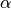from leapfrog steps.
• epsilon::Float64 : updated value of the step size parameter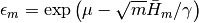if m > 0, and the user-supplied value otherwise.
• epsbar::Float64 : dual averaging parameter, defined as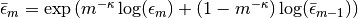.
• gamma::Float64 : dual averaging parameter, fixed at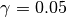.
• Hbar::Float64 : dual averaging parameter, defied as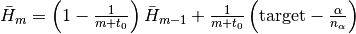.
• kappa::Float64 : dual averaging parameter, fixed at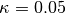.
• m::Int : number of adaptive update iterationsthat have been performed.
• mu::Float64 : dual averaging parameter, defined as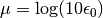.
• nalpha::Int : the total number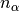of leapfrog steps performed.
• t0::Float64 : dual averaging parameter, fixed at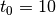.
• target::Float64 : target acceptance rate for the adaptive algorithm.